## New Maths Curriculum: Year 1 Fractions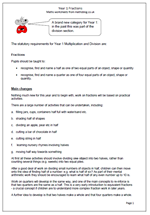Fractions is a brand new category for Year 1: in the past this was part of the division section.

The statutory requirements for Year 1 Multiplication and Division are:

Fractions

Pupils should be taught to:

•    recognise, find and name a half as one of two equal parts of an object, shape or quantity

•    recognise, find and name a quarter as one of four equal parts of an object, shape or quantity.

Main changes

Nothing much new for this year and to begin with, work on fractions will be based on practical activities.

There are a large number of activities that can be undertaken, including:

a.    filling jars, cups, containers half full with water/sand etc.

c.    dividing an apple, pear etc in half

d.    cutting a bar of chocolate in half

e.    cutting string in half

f.     learning nursery rhymes involving halves

g.    moving half way towards something

At first all these activities should involve dividing one object into two halves, rather than counting several things (e.g. sweets) into two equal piles.

After a good deal of work on dividing small numbers of objects in half, children can then move onto the idea of finding half of a number: e.g. what is half of six? As part of their mental arithmetic work they should be encouraged to learn what half of any even number up to 10 is.

Work on quarters will develop in the same way, and one of the main concepts to re-inforce is that two quarters are the same as a half. This is a very early introduction to equivalent fractions – a crucial concept if children are to understand more complex fraction work in later years.

A further idea to develop is that two halves make a whole and that four quarters make a whole.

Year 1 Fractions: statutory requirements

## New Maths Curriculum: Year 1 Multiplication and Division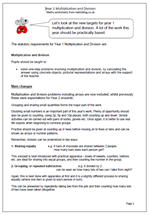Let’s look at the new targets for year 1 multiplication and division. A lot of the work this year should be practically based.

The statutory requirements for Year 1 Multiplication and Division are:

Multiplication and division

Pupils should be taught to:

•    solve one-step problems involving multiplication and division, by calculating the answer using concrete objects, pictorial representations and arrays with the support of the teacher.

Main changes

Multiplication and division problems including arrays are now included, whilst previously these were expectations for Year 2 onwards.

Grouping and sharing small quantities forms the major part of the work.

Doubling small numbers is an important part of this year’s work. Plenty of opportunity should also be given to counting, using 2p, 5p and 10p pieces, both counting up and down. Similar activities can be carried out with pairs of socks, gloves etc. Once again, it is better to use real life objects when beginning to combine groups.

Practice should be given at counting up in twos before moving on to fives or tens and can be shown as arrays or number patterns.
Remember, division can be understood in two ways:

1. Sharing equally               e.g. 6 bars of chocolate are shared between 2 people.
How many bars does each person get?

This concept is best introduced with practical apparatus – bowls of sweets, counters, buttons etc. are ideal for sharing into equal groups, and then counting the number in the group.

2. Grouping, or repeated subtraction                     e.g. 8 divided by 2
can be seen as how many lots of two can I take from eight?

Again, this is best done with apparatus at first and it is a slightly different process to sharing equally (where one item is given to each person in turn).

This can be answered by repeatedly taking two from the pile and then counting how many lots of two have been taken altogether.

Multiplication and division in Year 1

## New Maths Curriculum: Year 1 Addition and Subtraction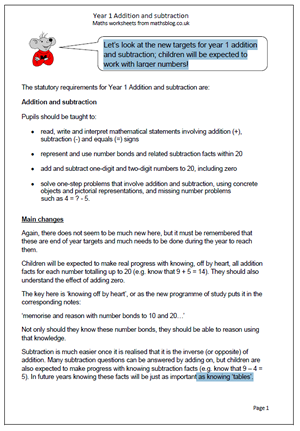Let’s look at the new targets for year 1 addition and subtraction; children will be expected to work with larger numbers!

Pupils should be taught to:

•    read, write and interpret mathematical statements involving addition (+), subtraction (-) and equals (=) signs

•    represent and use number bonds and related subtraction facts within 20

•    add and subtract one-digit and two-digit numbers to 20, including zero

•    solve one-step problems that involve addition and subtraction, using concrete objects and pictorial representations, and missing number problems
such as 4 = ? – 5.

Again, there does not seem to be much new here, but it must be remembered that these are end of year targets and much needs to be done during the year to reach them.
Children will be expected to make real progress with knowing, off by heart, all addition facts for each number totalling up to 20 (e.g. know that 9 + 5 = 14). They should also understand the effect of adding zero.
The key here is ‘knowing off by heart’, or as the new programme of study puts it in the corresponding notes:
‘memorise and reason with number bonds to 10 and 20…’
Not only should they know these number bonds, they should be able to reason using that knowledge.
Subtraction is much easier once it is realised that it is the inverse (or opposite) of addition. Many subtraction questions can be answered by adding on, but children are also expected to make progress with knowing subtraction facts (e.g. know that 9 – 4 = 5). In future years knowing these facts will be just as important as knowing ‘tables’.

New programme of Study: Year 1 Addition and Subtractionddition and subtraction

## New Maths Curriculum: Year 1 Number and Place Value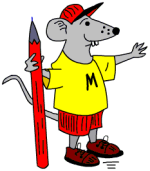It’s a whole new set of targets for Year 1 from 2014. Let’s begin by having a look at Number and Place Value.

The statutory requirements for Year 1 Number and Place Value are:

Number and place value

Pupils should be taught to:

•    count to and across 100, forwards and backwards, beginning with 0 or 1, or from any given number

•    count, read and write numbers to 100 in numerals; count in multiples of twos, fives and tens

•    given a number, identify one more and one less

•    identify and represent numbers using objects and pictorial representations including the number line, and use the language of: equal to, more than, less than (fewer), most, least

•    read and write numbers from 1 to 20 in numerals and words.

Main changes

The new maths programme of study is much shorter than previous models with much broader statements. This makes it tricky to know what has been left out. However, the main aim is to raise standards by raising targets to be achieved. In Year 1 the main changes are:

1. Counting, reading and writing numbers to 100 rather than just up to 20. This will prove to be very difficult for many children and will need a great deal of practice.
2. Perhaps even harder is to count in twos, fives and tens from different multiples.

New Statutory Requirements 2014:  Number and Place Value

## Year 6 resource of the week: factors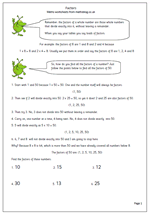Understanding factors and multiples is something many children fail to grasp. Here we have a page on factors and how to find all the factors of a number. The process is fairly straightforward, but can be quite time consuming. A good knowledge of tables and division is also needed. So if children’s knowledge of tables is weak, if they find division difficult and if they have little staying power then it is unlikely that they will enjoy trying to find factors of numbers!

One point that even brighter children take a while to understand is that you only need to continue to divide up to when the number squared is larger than the original number eg to find the factors of 62 you only need to divide 62 by numbers up to 7 because eight eights are 64. It is a good idea to spend some time explaining and showing the logic of this to children.

This can be found in our Year 6 Understanding Number section.

Factors 1

## Resource of the Week: Year 5 Number Challenge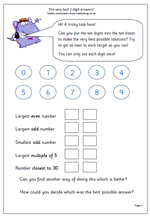I really like this challenge, partly because there is no, one right way to answer it and partly because it really makes children think.

There are 10 digits, from zero to nine to be placed in the 10 boxes in such a way that the targets can be matched as closely as possible. The catch is that each number can only be used once!

Now the obvious way to start is to make 98 the largest even number, but immediately that means that you can not have 97 as the largest odd number. By the time you reach the last target, number closest to 30, you have only two digits left and only two choices!

But what counts as the best possible answers? This is as big a challenge, if not bigger. I have had a class try to make a set of rules to try to be as fair as possible, but it involved a great deal of addition and subtraction. One group made a set of rules that went like this:

1. Find the difference between 98 and the answer given.

2. Find the difference between 99 and the answer given.

This will give your total so far – the larger the total, the worse you have done.

I won’t continue with this as it might spoil the fun!

This page can be found in our Year 5, Using and Understanding Maths category.

## Subtracting mentally in Year 4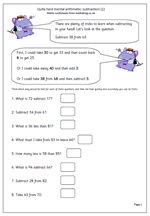Making the correct choice in deciding how to work out an answer is the key to fast mental arithmetic. Many children are unaware that there are often several ways of working out a calculation and that those who choose the best methods find maths easier and are able to answer questions more quickly and more accurately.

Let’s look at ‘Subtract 38 from 63.

This should be done, ‘in your head’ and will involve several stages, depending on the method used.

First, I could take 30 from 63 to leave 33. Then I could count back 8 from 33 which gives me 25.

Secondly, I could take 40 from 63 leaving 23 and then compensate by adding 2, which gives me 25.

Thirdly, I could take 38 from 68, leaving 30 and then compensate by subtracting 5 (because 63 is 5 less than 68).

There are several other ways very similar to these, but this does show that there is no ‘one right way, when it comes to mental arithmetic.

Quite hard mental arithmetic:_subtraction (1)

## Mental division practice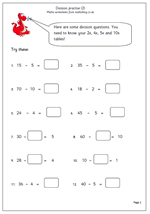being able to divide mentally is essential and is made much easier by knowing times tables.

Here is a follow up page to one published earlier, giving more practice with simple division. If children have a good knowledge of the 2x, 4x, 5x and 10x tables they should find these quite straightforward. The only potentially tricky ones are where the missing number is in the middle of the number sentence

eg 24 divided by ? = 6

This requires a good understanding of what the number sentence means, but all that is required to answer is a knowledge of ‘what multiplied by 6 makes 24’.

This worksheet can be found in our Year 3 Knowing Number Facts section.

Y3 division practice 2

## Pairs of numbers that make 20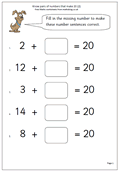Here we have a straightforward maths worksheet on knowing pairs of numbers that add up to twenty. This is suitable for year 1/2 children or those who are already very confident with knowing pairs of numbers that make ten.

If these facts are not known there are several ways of working the answers out, including:

1. Counting on from the smaller number.

2. Counting on from the smaller number up to 10 and then adding another 10. (If smaller number is below 10.)

3. Counting back from 20, which is trickier.

Know pairs that make 20 (pg 2)

## Resource of the week: year 1 investigate addition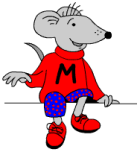Welcome to a new term and plenty of new maths material coming up over the next few months.

From our current resources here is a nice little investigation for young children which will show how well they can organise their thinking and work in a logical way.

The question is simple: how many different ways can you score 8 when throwing two dice?

It’s always a good idea to sit down with your children when doing this type of activity. In this way you can ask questions which will help them clarify their thoughts. The sheet is designed to be used as a record of results. At first they might just keep rolling the dice and adding up the totals until a total of 8 is achieved. Other children might dive straight in with some answers eg 4 + 4 makes 8.

A good question to ask at the end is “How do you know that you have got all the possibilities?

Free Y1 maths worksheet: Investigate dice